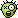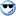PHP User Warning: fetch_template() calls should be replaced by the vB_Template class. Template name: bbcode_highlight in ..../includes/functions.php on line 4197
Select and move multiple movieclips dynamically (AS2, CS4) - Page 2
A Flash Developer Resource Site

# Thread: Select and move multiple movieclips dynamically (AS2, CS4)

1. That's a great find.I'd been looking through this thread

but the "Dynamic MovieClip Registration with AS2" by darronschall was great and easy to use... Thanks!

Actionscript Code:
`import com.darronschall.DynamicRegistration;import mx.transitions.Tween;// Import tween class import mx.transitions.easing.*;// Import easing class // Assume there is an instance named square_mc on the stage  var square_mc:MovieClip;    DynamicRegistration.initialize(square_mc);  // set up initial registration pointvar centerX = square_mc._width / 2;var centerY = square_mc._height / 2;square_mc.setRegistration(centerX,centerY);var rotateMe = 90;my_butt.onRelease = function() {     my_butt.enabled = false;     var thisTween:Tween = new Tween(square_mc, "_rotation2", Regular.easeOut, square_mc._rotation2, rotateMe, 1, true);      thisTween.onMotionFinished = function() {         delete this;         rotateMe += 90;        if(rotateMe > 270) {            rotateMe = 0;        }        trace("rotateMe = " + rotateMe);        my_butt.enabled = true;     };  };`

Now, back to the problem of rotating (or flipping) more than one selected item...Reply With Quote

2. ...Reply With Quote

3. Wow - that's awesome...
Thanks to both of you...!

Dare I ask - how would you then flip (ie reflect around centerpoint) the grouped items?Reply With Quote

4. ...Reply With Quote

5. Just wow. Thanks indeed!

Flashkit (and all who sail in her) for president...Reply With Quote

6. hmmm...

there is a strange behaviour on flipping. Set the rotation to 90 degrees. Select more than one object and rotate once, then flip grouped objects, and you'll see what I mean...
Any ideas?

Also, how would you set-up the background as a button so that if you clicked on it, it deselected all the objects. Sounds easy, but I can't figure it out...

Thanks!Reply With Quote

7. Not seeing the problem, can you post pics?

...Reply With Quote

8. Nice fix on the background click! I was going in the wrong direction on that one, using an invisible button...

Here are pics:
screenshot_01.png
screenshot_02.png
screenshot_03.png
screenshot_04.png

The first shows configuration of objects
Second demonstrates correct flip.
Third shows objects rotated 90 degrees.
Fourth shows incorrect flip.

Flip button used is top one on mc.

Hope this makes sense.

Cheers!Reply With Quote

9. ...Reply With Quote

10. Brilliant. You make it look easy. Now I'm trying to add a tween to the translations... Is that even possible?

Thanks!Reply With Quote

11. ...Reply With Quote

12. dawsonk!That is better than poetry! Thank you so very much...Reply With Quote

13. Hi again.

Just noticed a very strange anomaly. Everything works brilliantly. However, if you select the 1 shape and the 2 shape (in version below) and press rotate, it incorrectly rotates the objects onto one another. I can't for the life of me figure out why...

Any ideas?Reply With Quote

14. Code:
```import flash.filters.DropShadowFilter;
import flash.geom.Point;
import mx.transitions.Tween;
import mx.transitions.easing.*;

var rotationAmt = 90;
var x1, y1, x2, y2;
var pickedArray = new Array();

// movie clips on the stage, registration points are in the center
shape1001.onPress = doPress;
shape1002.onPress = doPress;
shape1003.onPress = doPress;
shape1004.onPress = doPress;
shape1005.onPress = doPress;
shape1006.onPress = doPress;

// movie clips on stage, registration point are in the center
rotatorMC._visible = false;
flipperMC._visible = false;

rotatorMC.onPress = doRotate;
flipperMC.onPress = doFlip;
rotateButton.onPress = doRotate;
flipButton.onPress = doFlip;

var bg = this;
bg.onMouseDown = clearPicked;
var intID;
var isLocked = false;

function setLimit() {
isLocked = true;
intID = setInterval(clearLimit, 600);
}
function clearLimit() {
clearInterval(intID);
isLocked = false;
flipButton.enabled = true;
rotateButton.enabled = true;
}

function clearPicked() {
var isPicked = false;
for (var j in bg) {
if (typeof (bg[j]) == "movieclip" && bg[j] != this) {
if (bg[j].hitTest(_xmouse, _ymouse, true)) {
isPicked = true;
break;
}
}
}
if (!isPicked) {
for (var i in pickedArray) {
pickedArray[i].filters = null;
pickedArray[i].swapDepths(pickedArray[i].getDepth() * -1);
}
pickedArray = new Array();
updatePos(true);
}
}

function doFlip() {
if (!isLocked) {
flipButton.enabled = false;
flipButton.gotoAndStop(1);
setLimit();
var isV = (rotatorMC._y>_ymouse) ? true : false;
for (var i in pickedArray) {
var mc = pickedArray[i];
if (isV) {
if (Math.abs(mc._rotation) != 90) {
new Tween(mc, "_xscale", Strong.easeOut, mc._xscale, -mc._xscale, 0.5, true);
} else {
new Tween(mc, "_xscale", Strong.easeOut, mc._xscale, 100, 0.5, true);
new Tween(mc, "_yscale", Strong.easeOut, mc._yscale, -mc._yscale, 0.5, true);
}
new Tween(mc, "_x", Strong.easeOut, mc._x, rotatorMC._x+(rotatorMC._x-mc._x), 0.5, true);
} else {
if (Math.abs(mc._rotation) != 90) {
new Tween(mc, "_yscale", Strong.easeOut, mc._yscale, -mc._yscale, 0.5, true);
} else {
new Tween(mc, "_yscale", Strong.easeOut, mc._yscale, 100, 0.5, true);
new Tween(mc, "_xscale", Strong.easeOut, mc._xscale, -mc._xscale, 0.5, true);
}
new Tween(mc, "_y", Strong.easeOut, mc._y, rotatorMC._y+(rotatorMC._y-mc._y), 0.5, true);
}
}
}
}

function doRotate() {
if (!isLocked) {
rotateButton.enabled = false;
rotateButton.gotoAndStop(1);
setLimit();
var cen = rotatorMC;
var dir = (cen._x>_xmouse) ? -1 : 1;
var p1:Point = new Point(cen._x, cen._y);
for (var i in pickedArray) {
var mc = pickedArray[i];
var p2 = new Point(mc._x, mc._y);
var len = Point.distance(p1, p2);
var angle;
if (cen._y>mc._y) {
angle = Math.round(Math.atan((cen._y-mc._y)/(cen._x-mc._x))*(180/Math.PI));
angle = (angle<0) ? 180+angle : angle;
} else if (cen._y<mc._y) {
angle = Math.round(Math.atan((mc._y-cen._y)/(mc._x-cen._x))*(180/Math.PI));
angle = (angle>=0) ? 180+angle : angle;
} else {
trace(cen._x+" "+mc._x)
angle = (cen._x<mc._x) ? -180 : 360;
}
new Tween(mc, "_rotation", Strong.easeOut, mc._rotation, mc._rotation+(90*dir), 0.5, true);
new Tween(mc, "_x", Strong.easeOut, mc._x, cen._x-(len*Math.cos((angle+(90*dir))*Math.PI/180)), 0.5, true);
new Tween(mc, "_y", Strong.easeOut, mc._y, cen._y-(len*Math.sin((angle+(90*dir))*Math.PI/180)), 0.5, true);
}
}
}

function doPress() {
var isPicked = true;
for (var i in pickedArray) {
if (pickedArray[i] == this) {
pickedArray.splice(i,1);
isPicked = true;
this.filters = null;
updatePos(true);
this.swapDepths(this.getDepth() * -1);
break;
}
}
if (isPicked) {
var filter = new DropShadowFilter(3, 45, 0x000033, 0.45, 5, 5, 1, 3, false, false, false);
var filterArray = new Array();
filterArray.push(filter);
this.filters = filterArray;
pickedArray.push(this);
updatePos(true);
this.swapDepths(pickedArray.length);
this.onMouseMove = updatePos;
this.onMouseUp = clearMouse;
}
}
function updatePos(isSet) {
x1 = Stage.width;
y1 = Stage.height;
x2 = 0;
y2 = 0;
for (var j in pickedArray) {
if (isSet != undefined) {
pickedArray[j].baseX = pickedArray[j]._x - _xmouse;
pickedArray[j].baseY = pickedArray[j]._y - _ymouse;
} else {
pickedArray[j]._x = pickedArray[j].baseX + _xmouse;
pickedArray[j]._y = pickedArray[j].baseY + _ymouse;
}
pickedArray[j]._x = Math.round(pickedArray[j]._x);
pickedArray[j]._y = Math.round(pickedArray[j]._y);
x1 = Math.min(x1, pickedArray[j]._x);
y1 = Math.min(y1, pickedArray[j]._y);
x2 = Math.max(x2, pickedArray[j]._x);
y2 = Math.max(y2, pickedArray[j]._y);
}
rotatorMC._x = flipperMC._x = ((x2 - x1) / 2) + x1;
rotatorMC._y = flipperMC._y = ((y2 - y1) / 2) + y1;
rotatorMC.swapDepths(pickedArray.length + 10);
flipperMC.swapDepths(pickedArray.length + 9);
rotatorMC._visible = flipperMC._visible = (pickedArray.length > 0) ? false : false;
}

function clearMouse() {
delete this.onMouseMove;
delete this.onMouseUp;
}```Reply With Quote

15. Just two lines of code? Easy when you know howThanks a million!

How would you scale the selected group by 25% increments, bigger or smaller?

Also, there is an odd behaviour with the objects on different layers...
1. Select one object and move it over another one.
2. Then select the object that is below the first one, and move it so that it rises above the first one.
3. When you drop it, it falls behind the first object, rather than remain at the front.

??Reply With Quote

16. Hello again. I found a way to do the scalingAny ideas on the depth problem I mentioned above. I need the last selected object to be on top of all others....

Any pointers much appreciated.Reply With Quote

17. Code:
```function clearPicked() {
var isPicked = false;
for (var j in bg) {
if (typeof (bg[j]) == "movieclip" && bg[j] != this) {
if (bg[j].hitTest(_xmouse, _ymouse, true)) {
isPicked = true;
break;
}
}
}
if (!isPicked) {
for (var i in pickedArray) {
pickedArray[i].filters = null;
//pickedArray[i].swapDepths(pickedArray[i].getDepth()*-1);
}
pickedArray = new Array();
updatePos(true);
}
}

function doPress() {
var isPicked = true;
for (var i in pickedArray) {
if (pickedArray[i] == this) {
pickedArray.splice(i,1);
isPicked = true;
this.filters = null;
updatePos(true);
//this.swapDepths(this.getDepth()*-1);
break;
}
}
if (isPicked) {
var filter = new DropShadowFilter(3, 45, 0x000033, 0.45, 5, 5, 1, 3, false, false, false);
var filterArray = new Array();
filterArray.push(filter);
this.filters = filterArray;
pickedArray.push(this);
updatePos(true);
//this.swapDepths(pickedArray.length);
this.swapDepths(bg.getNextHighestDepth());
this.onMouseMove = updatePos;
this.onMouseUp = clearMouse;
}
}```Reply With Quote

18. Perfect...

I was very close to getting that one!

Thanks indeedReply With Quote

array, multiple, objects####Posting Permissions

• You may not post new threads
• You may not post replies
• You may not post attachments
• You may not edit your posts
•

 » Home » Movies » Tutorials » Submissions » Board » Links » Reviews » Feedback » Gallery » Fonts » The Lounge » Sound Loops » Sound FX » About FK » Sitemap